﻿ 基于多层共享备用路由器的多层网络恢复 The Restoration of Multilayer Optical Network Based on Multilayer Shared Backup Router

Software Engineering and Applications
Vol.06 No.05(2017), Article ID:22212,8 pages
10.12677/SEA.2017.65011

The Restoration of Multilayer Optical Network Based on Multilayer Shared Backup Router

Yuan Tang1, Hanhua Huang1, Ye Jiang1, Xiaomei Wang2

1Electric Power Dispatch & Control Center, Guangxi Power Grid Co., Ltd, Nanning Guangxi

2State Key Laboratory of Networking and Switching Technology, Beijing University of Posts and Telecommunications, Beijing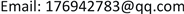Received: Sep. 8th, 2017; accepted: Sep. 22nd, 2017; published: Sep. 28th, 2017ABSTRACT

The current network is composed of optical transport network and IP network, and in the multilayer network, network resources protection and recovery mechanism is the concern of operators. In this paper, based on the multilayer optical network architecture, a method of multilayer shared backup router is proposed to realize the protection and restoration of network resources. In the aspect of cost, compared with the traditional multilayer network protection mechanism, the final result shows that the proposed scheme has a better performance than the traditional scheme.

Keywords:Multilayer Optical Network, Network Restoration, Network Protection, CAPEX, Multilayer Shared Backup Router, Availability

1广西电网公司电力调度控制中心，广西 南宁

2北京邮电大学网络与交换技术国家重点实验室，北京1. 引言Figure 1. Multi-layer optical network basic architecture

MLSBR方法的关键是使用额外的多层共享备用路由器，取代双平面保护中大量的闲置路由器，在出现网络故障时，可以重新计算路径将流量引入备用路由器从而恢复中断的网络，这和传统的恢复机制对比，可以极大降低运营商架构网络时的成本。

2. 相关工作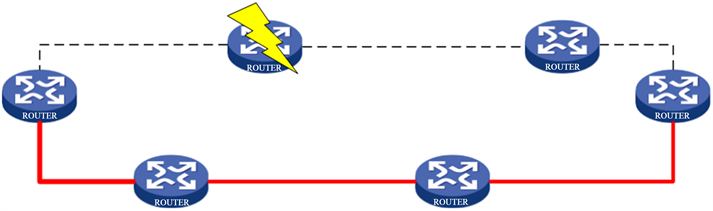Figure 2. Double plane protection

3. 共享备用路由以及案例分析

1) 发生故障。

2) 丢失连接的IP/MPLS节点向周围发送请求建立新的连入网络的路径。此时有两种情况。

a) 本地区域备用路由器可用时，通过请求备用路由器重新建立路径。

b) 本地区域备用路由器不可用时，通过请求连入临近区域的备用路由器重新建立路径。

3) 本地区域备用路由或邻近区域备用路由收到请求，检查自身工作状态，并发送相应的反馈信息。

4) IP/MPLS节点接收备用路由器的反馈信息选择建立新路径或是请求其他备用路由器。如果需要请求其他备用路由器，则返回第二步继续循环。反之，完成备用路径的建立。

5) 故障路由器修复后，释放建立的备用路径，将流量重新引入工作路径。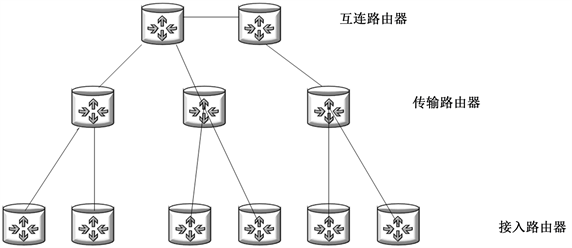Figure 3. IP layered architecture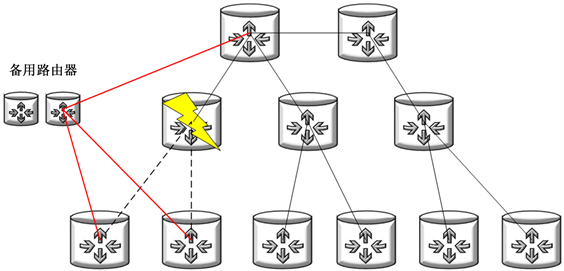Figure 4. Share backup route recovery mechanism

4. 可用性对比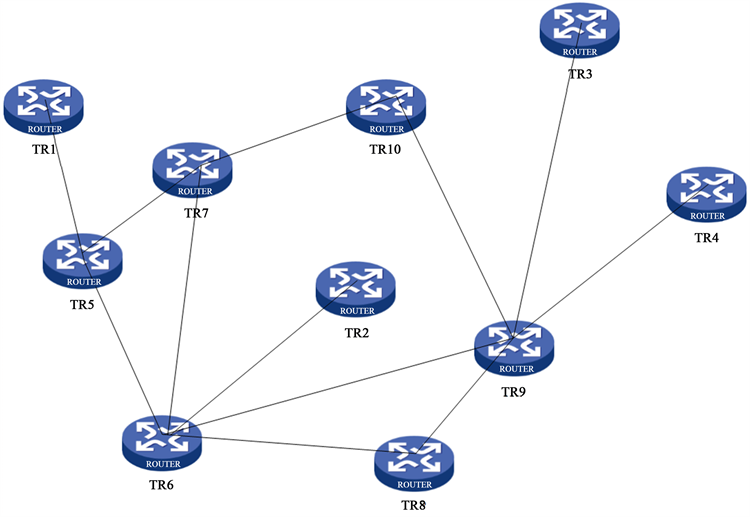Figure 5. IP topology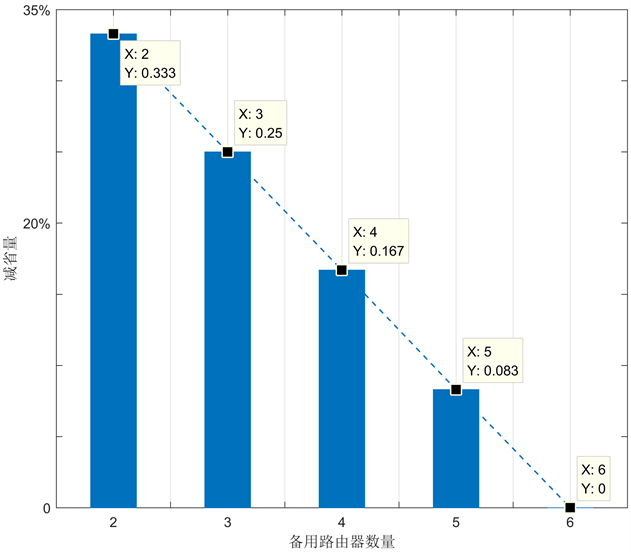Figure 6. The number of backup routers and the amount of reduction

$\text{Ava}=1-\frac{停机时间}{运行时间}$ (1)

${E}_{\text{Ava}}=\text{}\frac{\text{MTBF}}{\text{MTBF}+\text{MTTR}}$ (2)

${E}_{\text{Un}}=1-{E}_{\text{Ava}}=1-\frac{\text{MTBF}}{\text{MTTR}+\text{MTBF}}=\frac{\text{MTTR}}{\text{MTTR}+\text{MTBF}}$ (3)

${E}_{\text{Un}}=\frac{\lambda }{\lambda +1}$ (4)

4.1. 单区域

$\text{Ava}=1-{E}_{\text{Un}}^{2}=1-{\left(\frac{\lambda }{\lambda +1}\right)}^{2}$ (5)

4.2. 双区域

$\text{Ava}=1-{E}_{\text{Un}}^{2}=1-{\left(\frac{\lambda }{\lambda +1}\right)}^{2}$ (6)

$\text{Ava}=1-{E}_{\text{Un}}^{4}-{E}_{\text{Un}}^{3}{E}_{\text{Ava}}=1-{\left(\frac{\lambda }{\lambda +1}\right)}^{4}-{\left(\frac{\lambda }{\lambda +1}\right)}^{3}\frac{1}{\lambda +1}=1-\frac{{\lambda }^{4}+{\lambda }^{3}}{{\left(\lambda +1\right)}^{4}}$ (7)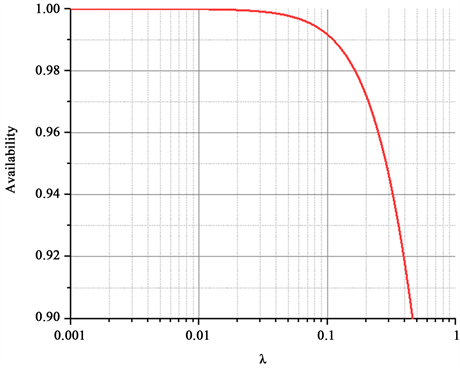Figure 7. The availability of both methods in a single region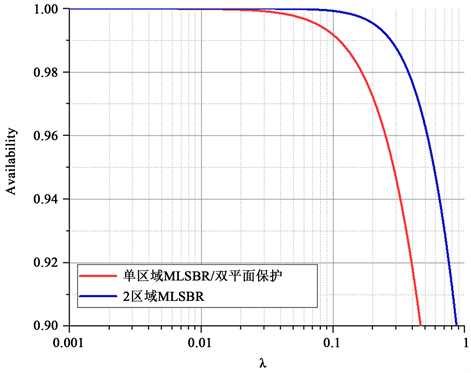Figure 8. The availability of two methods in double regions

5. 结束语

The Restoration of Multilayer Optical Network Based on Multilayer Shared Backup Router[J]. 软件工程与应用, 2017, 06(05): 103-110. http://dx.doi.org/10.12677/SEA.2017.65011

1. 1. 刘珂. 基于线性规划的大规模网络故障恢复机制[J]. 计算机工程, 2016, 42(7): 104-108+116.

2. 2. 曹毅宁, 谢永强, 许波, 等. IP/WDM网络的跨层控制系统设计[J]. 计算机工程, 2014, 40(9): 87-91.

3. 3. 曹毅宁, 郑小平, 李艳和, 等. IP/WDM网络的虚拓扑设计[J]. 计算机工程, 2010, 36(5): 4-6, 9.

4. 4. 孙罡. 多层网络生存性研究[D]: [硕士学位论文]. 成都: 成都理工大学, 2009.

5. 5. 邱强. IP over WDM网络生存性研究[D]: [硕士学位论文]. 北京: 北京邮电大学, 2009.

6. 6. Lee, K., Modiano, E. and Lee, H.W. (2011) Cross-Layer Survivability in WDM-Based Networks. IEEE/ACM Transactions on Networking, 19, 1000-1013. https://doi.org/10.1109/TNET.2010.2091426

7. 7. 李书, 陶君, 张丹. 软件定义网络中链路故障恢复机制研究[J]. 信息通信, 2016(12): 20-21.

8. 8. 王建国, 景鑫, 温海鹏. 基于BFD的MPLS网络自愈恢复技术的研究与实现[J]. 中国新通信, 2016, 18(15): 43.

9. 9. Palkopoulou, E., Schupke, D.A. and Bauschert, T. (2011) Shared Backup Router Resources: Realizing Virtualized Network Resilience. IEEE Communications Magazine, 49, 140-146. https://doi.org/10.1109/MCOM.2011.5762810

10. 10. Muñoz, F., López, V., González de Dios, Ó., et al. (2012) Multi-Layer Restoration in Hierarchical IP/MPLS over WSON Networks. In: European Conference on Networks and Optical Communications (NOC), IEEE Press, Washington DC, 1-6.

11. 11. Mayoral, A., López, V., Gerstel, O., et al. (2014) Minimizing Resource Protection in IP over WDM Networks: Multi-Layer Shared Backup Router. In: Proceedings of Optical Fiber Conference (OFC), IEEE Press, Washington DC, 1-3. https://doi.org/10.1364/OFC.2014.M3B.1

12. 12. 李燕伟, 华楠, 陈悦, 等. 多层生存性网络层间协调机制建模及实验评估[J]. 清华大学学报, 2013(3): 399-403.